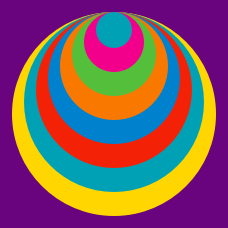Geometry

# Composite Figures: Level 3 Challenges

The 2 green points in the diagram are the midpoints of 2 adjacent sides of a regular hexagon.

Which is larger, the red area or the blue area?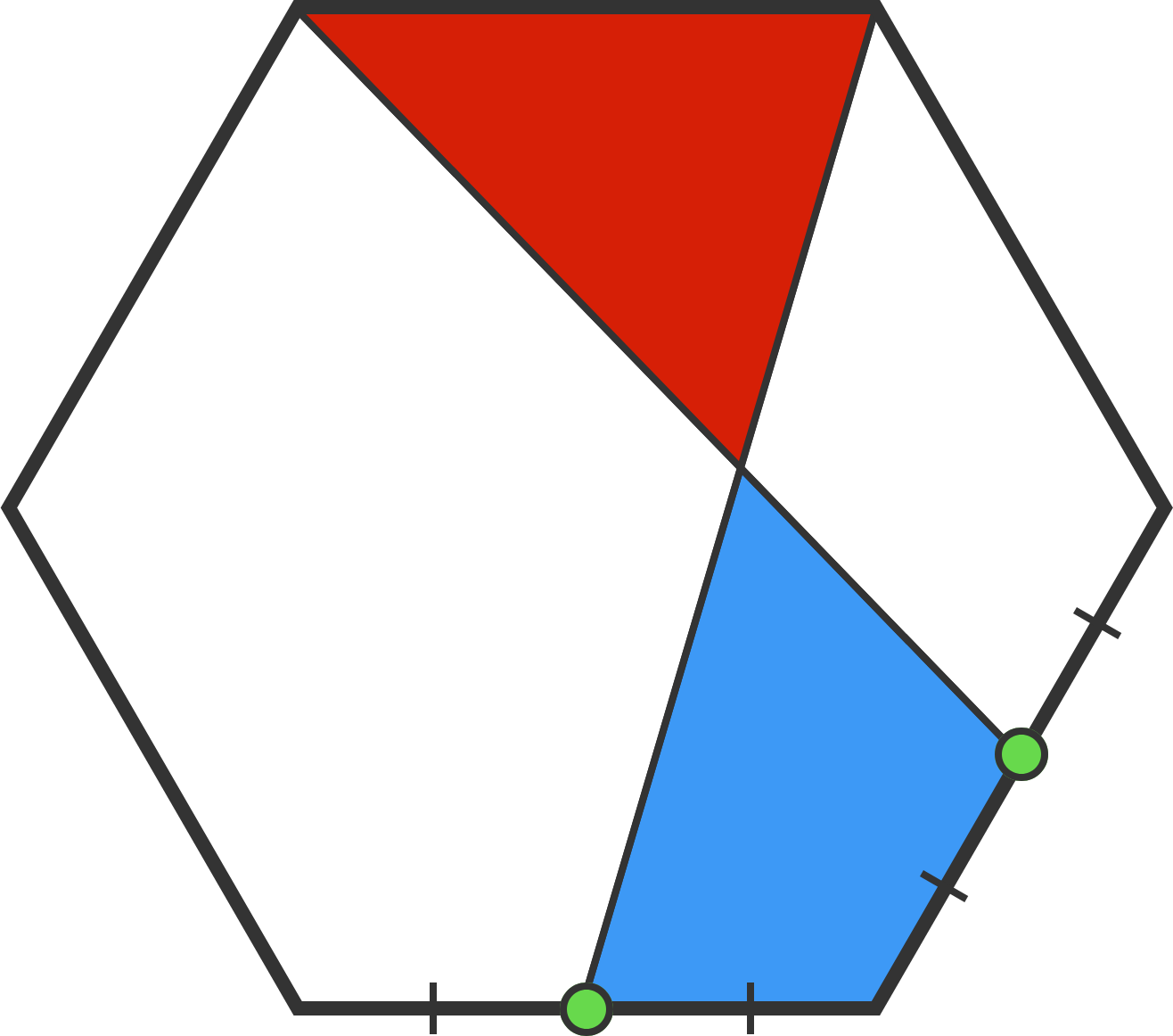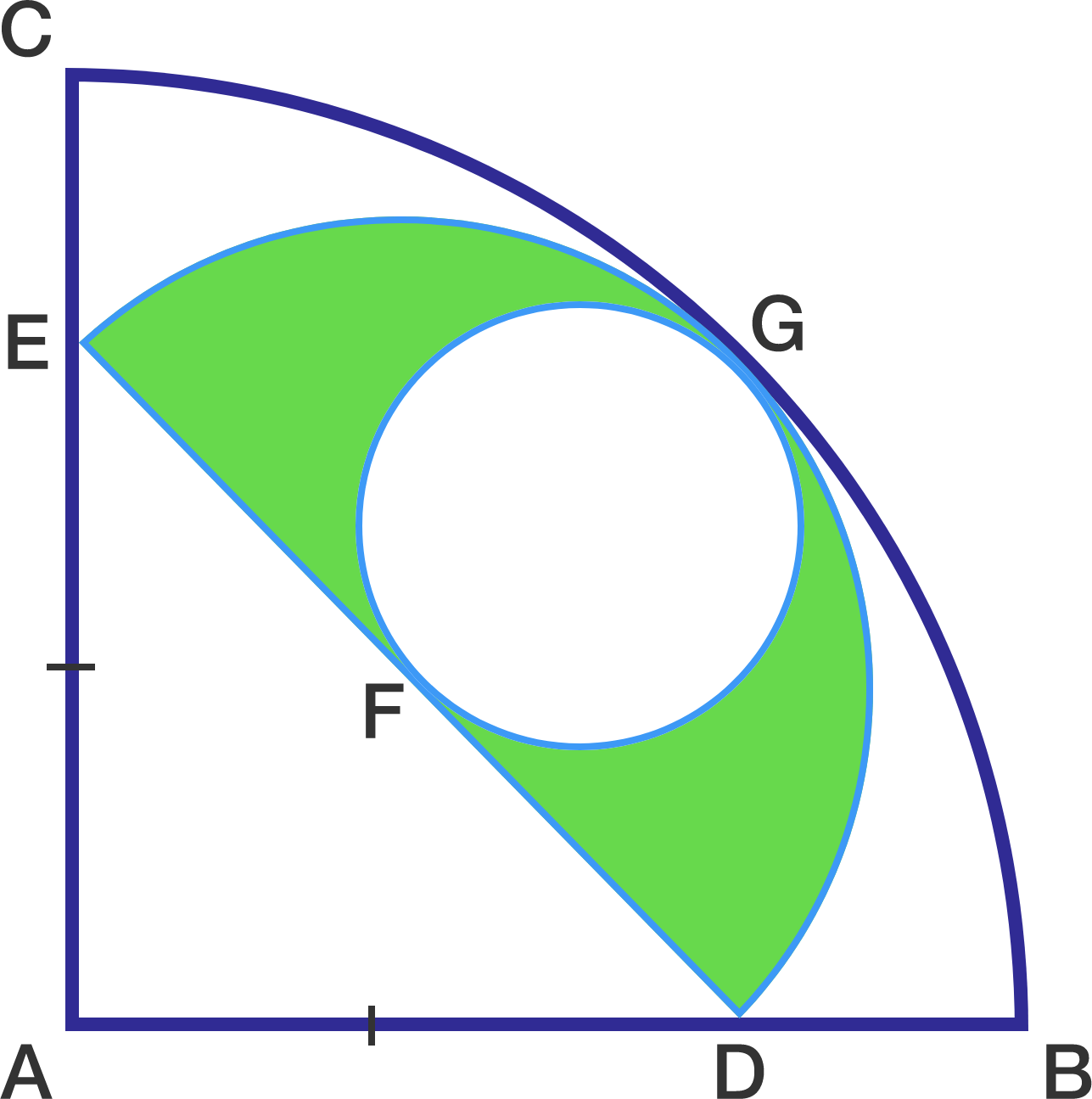The diagram above shows that a semicircle is inscribed in a quarter circle while a small circle is inscribed in the semicircle. Given that $AD=AE$ and the radius of the quarter circle is $14\sqrt{2} \text{ cm}$, find the area of the green region above (in $\text{cm}^{2}$).

For your final step, use the approximation $\pi = \dfrac{22}{7}$.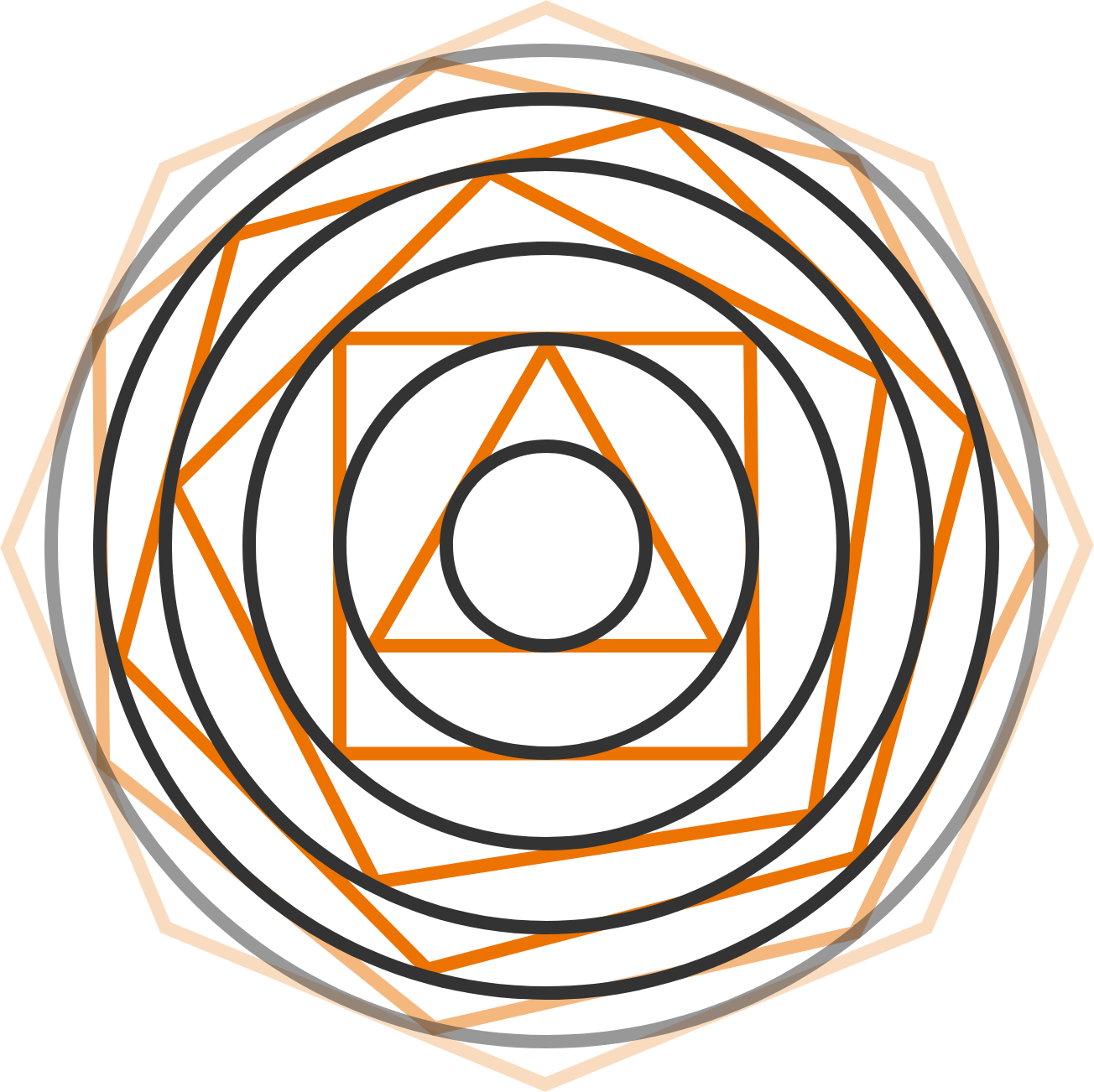I begin with a circle of radius $1 \text{ cm}$, which becomes the inscribed circle of an equilateral triangle, which I enclose with its circumcircle (the circle that touches all of its vertices). I enclose this circle with a square (so that each edge touches the circle once), and surround this with the square's circumcircle. I repeat this procedure with a pentagon, a hexagon, and so on forever (increasing the side number by one, until we reach an infinity-sided polygon).

What is the limiting area (in $\text{cm}^2$) of the "final" circle, rounded to a whole number?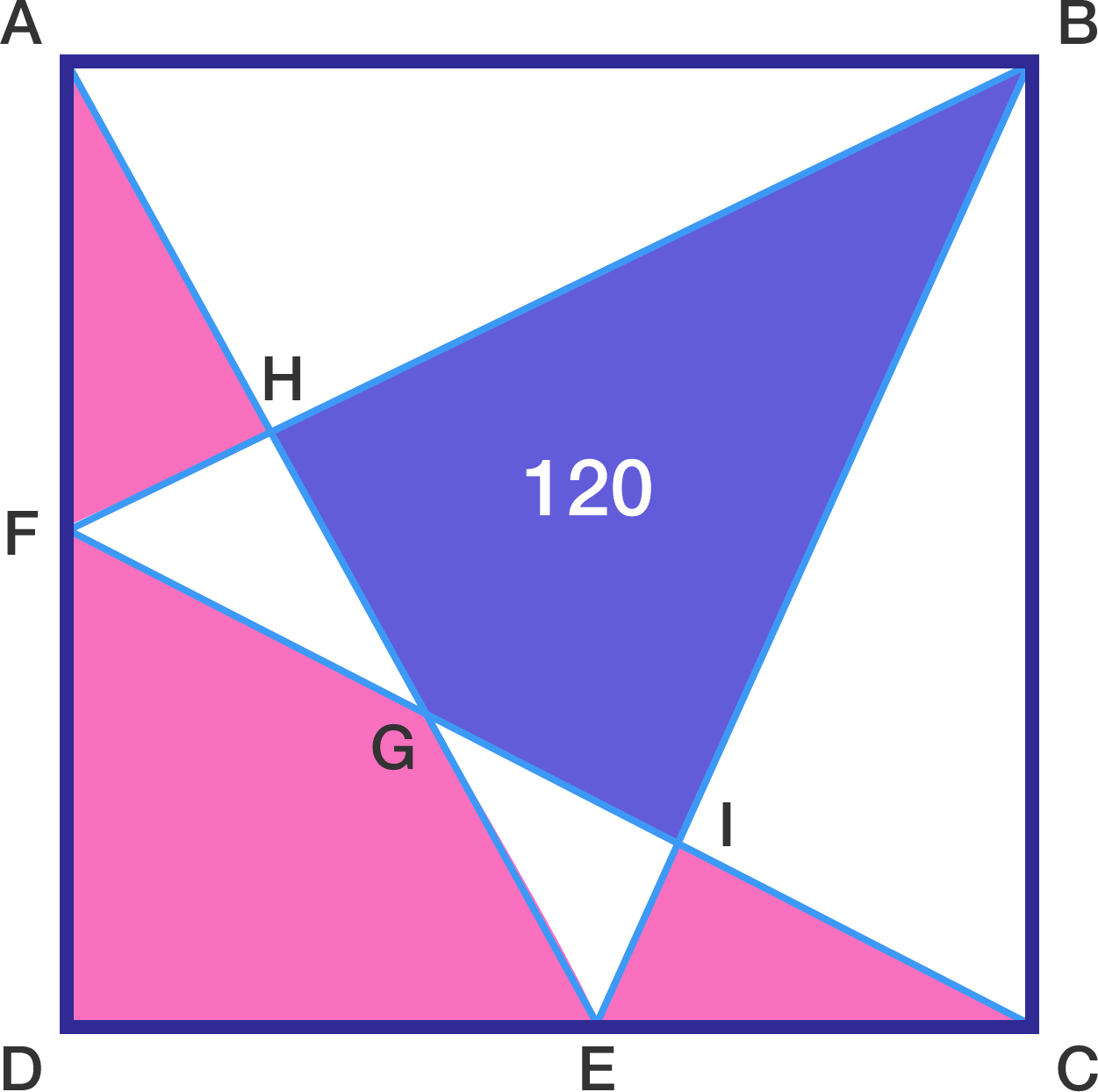$ABCD$ is a square with points $E$ and $F$ lying on sides $CD$ and $AD,$ respectively. If the purple area is $[BHGI]=120,$ what is the sum of the pink areas $[AHF]+[FGED]+[ICE]?$

What is the area of the large square?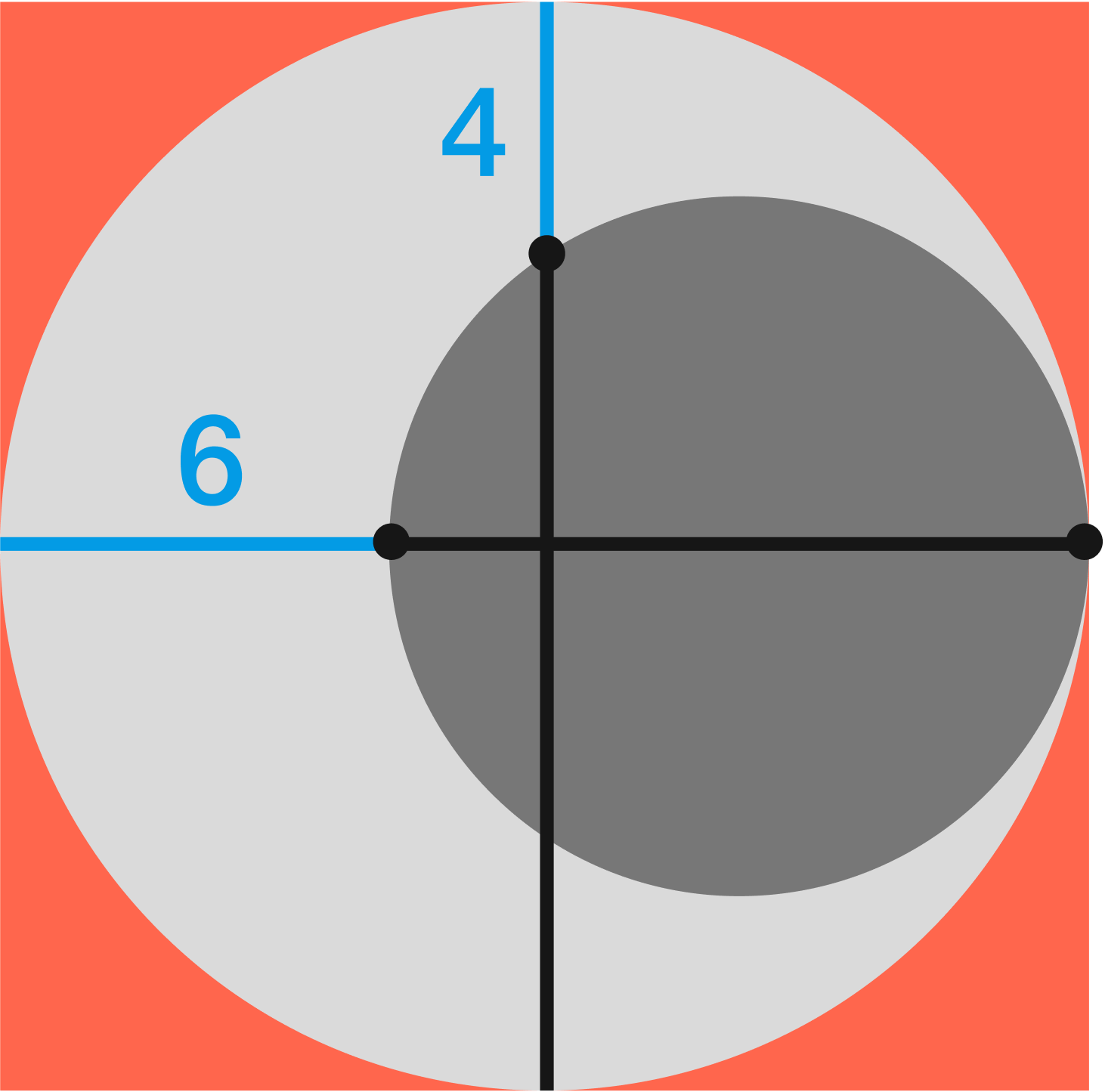×